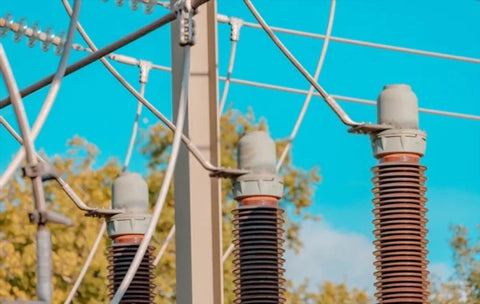Watts to kVA Calculator - ShopSolar.com

# Watts to kVA Calculator

Converting watts to kVA is fairly easy to do, but it can get confusing if you're unfamiliar with the different engineering terms and units of measurement involved. To get a better grasp of the subject, we also need to take a look at what exactly the term “kilovolt-amps” (kVA) means.

It doesn’t stop with watts to kVA though, you can also use our general watt calculator to help out with a variety of different solar system equations.

 Enter watts: W Enter power factor: Result in kilovolt-amps: kVA

## Converting Watts to kVA

To be able to convert watts to kVA, you first need to understand power factor.### Power Factor

The power factor of an electrical system refers to the ratio of watts/kilowatts to kVA, of real power to apparent power (we discuss these terms below).

It takes the form of a number between 0 and 1. For example, a good power factor is 0.8 or higher.

By determining the ratio of real power to apparent power within an electrical system, the power factor acts as a tool to measure the overall efficiency of the circuit. This is measured by how much energy is converted to do the work and how much dissipates due to resistance.

Bear in mind that watts aren’t the same as kilowatt hours, but you can convert one to the other. Have a look at our watts to kWh calculator to find out more.

It's important to be aware of this because in most cases, a poor power factor will leave you with a higher electricity bill.

The power factor (PF) of an electrical system can be calculated using the following formula:

PF = (Real Power) / (Apparent Power)

Or

PF = Watts ÷ VA

## How to Convert Watts to kVATo convert watts to kVA you have to divide the watts by 1,000 times the power factor. When written out, the formula will look something like this:

kVA = Watts/1,000 x Power Factor

As an example, let's find the apparent power in kVA of a 2,000-watt circuit with a power factor of 0.8:

kVA = 2000w ÷ (1,000 x 0.8)

kVA = 2.5 kVA

## What Is kVA?

kVA Is short for kilovolt-amperes. It’s a unit of measurement used to refer to electricity in the form of “apparent power” in an alternating current (AC) or direct current (DC) electrical circuit.

It’s measured as the product of the voltage, which represents electrical pressure, and the amperes (electrical current). For more, check out how to convert watts to amps.

In an alternating current circuit, the kVA or ‘apparent power’ is different from the ‘real power’ (expressed as watts or kilowatts), which goes into the circuit to perform its tasks. In a direct current electrical circuit, the kVA and kW values are equal since the voltage and load are static, and the energy only flows in one direction.

To fully understand what a kVA is, you first need to understand the different types of power in an electrical system, what’s known as “apparent power,” “real power,” and “reactive power.”

### Apparent Power

Apparent power is the sum of the power flowing within an electrical circuit. When electricity flows through an AC circuit, not all of the power generated is used with 100% efficiency.

In the case of solar power systems, apparent power is the total amount of energy generated by the system as a whole. This power is what we measure as kVA.

### Real Power

Real power, also known as true or working power, refers to the electricity in a circuit that’s actually being used and is doing the work to perform a specific task, like powering an appliance. The electricity used is generally measured in watts (w) or kilowatts (kW).

Watts and kilowatts are both a unit of measurement for electricity in the form of real power. Kilowatts express electricity in higher quantities, since 1 kilowatt is equal to 1,000 watts. In a solar panel system, the real power is the energy sent to and used by the household.

This is why it’s essential to work out your generator’s wattage.

### Reactive Power

Not all of the energy in an alternating current circuit is used to perform a function in the electrical system, and the energy that isn’t used is called “reactive power.”

Reactive power, also known as phantom power, within the context of a solar panel system is the electricity generated by the system that doesn’t get used by the household and is sent back to the grid instead. Reactive power is measured in volt-amperes reactive (VAR).

You can find all of the we have created in order to help you plan, size and install your own solar power system here.

## Join the Solar Lab!